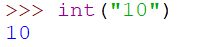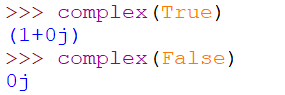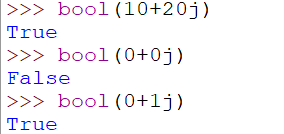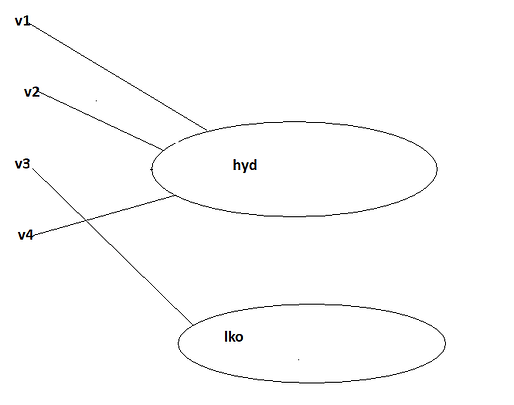top of page## Python: Types Casting

Type Casting in Python

Type casting is conversion of one data type to other data types.

There are some inbuilt function in Python to change one data type to another:

int()

float()

complex()

bool()

str()

1. int(): we can use int() to convert any other type (except complex) to int.

a. float to int

Type Castingb. complex to int: conversion is not possible, if you are trying to do so you will get syntax error.c. bool to intd. str to int: if you want to convert string to int, compulsorily the string should represent only int values. That int value must be in base 10 (decimal form).2. float(): we can use float() to convert any other type (except complex type) to float.

a. bool to float:b. complex to float conversion is not possible if you are trying to do so you will get syntax error.c. str to float: if you want convert string to float, compulsorily the string should represent only int values. That int value must be in base 10 (decimal form).3. complex(): to convert any data type to complex.

There are two forms for this function. These two functions are called overloaded functions, which means function name is same but no of argument  is different.

Form-1

complex(x)==>x+oj

If we are passing only one argument, it is considered as real part.

a. int to complexb. float to complexc. bool to complexd. str to complexForm-2

complex(x,y)==>x+yj

If we are passing two arguments, first argument is considered as real part and second argument is considered as imaginary part.

a.int to complexb.float to complexc. bool to complexd. str to complex: complex can not take second argument if first  is a string.4. bool(): to convert any data type to boolean.

a. for int argument

If argument is 0 then result is False

bool(0)==>False

If argument is non zero then result is True

bool(1)==>True

bool(2)==>True

bool(10)==>True

bool(-10)==>Trueb. for float argument

If argument is 0.0 then result is False

If argument is non zero then result is Truec. for complex argument

If both imaginary and real part is 0 then result is False.

If at least one part is non zero then result is True.d. for str argument

If argument is empty string  then result is False.

If argument is non empty string  then result is True.5. str(): to convert any data type to strings.All 5 fundamental data types are immutable

Immutable means non changeable. Once we create an object, we are not allowed to perform any change in the existing object. If we perform any change, a new object is created.

Why immutability concept is required in Python?

In python, same object is used by multiple references. By doing so performance and memory utilization is improved.

If we are trying to change the content using one reference then remaining references also gets affected. To prevent this immutability concept is introduced in python, so if we are trying to perform any change in the content, a new object is created with that changes.

For example if v1,v2,v3,v4 are four reference variables pointing to the same object with content 'hyd'.

ImmutabilityIf we want to change the content hyd to lko using v3 reference variable, a new object is created and v3 is pointing to this object with content ‘lko’is operator

Note 1: Everything in python is an object.

Note 2: All fundamental data types in python are immutable.

'is' operator in Python

'is' operator in Python is used to find whether references are pointing to the same object or not.

If answer is True  references are pointing to the same object.

If answer is False  references are not pointing to the same object.

For example if a=10 and b=10

a is b==>True

b is a==>Truea=10

b=20

a is b ==>False

b is a==>FalseNote 1:

• Reusing same object: such type of concept is defined only in the following ranges .

Int ==>0 to 256

bool ==>always

str ==>always

• This concept is not applicable for complex and float. In case of float and complex, always a new object is created.Note 2:  0 to 256 is the most commonly used range

When Python interpreter starts before your program execution, only from 0 to 256 integer object will be created. So at run time we are not required to create these objects separately, hence run type performance is improved automatically.

Note 3: bool contains only two values True and False and for this 2 objects will be created.

Note 4: str is most commonly used in our program, so reusing the object again and again is applicable for str type.

Why reusability concept is not for float and complex

• There are so many floating point values, even between 0 to 1 infinite floating values are there, so it is not possible to decide frequently used range for float.

• In case of complex type, it is not possible to define the most commonly used range because complex values may contain floating point values.

Note:

• int, float, complex, bool, str these five are considered as fundamental data types.

• All fundamental data types are immutable.

For any Query/Suggestion, do let us know in the comment section.

bottom of page